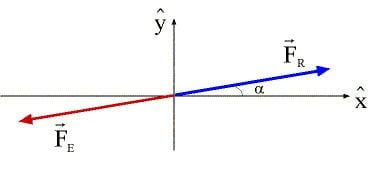# Equilibrant force

Updated on
Covid-19Equilibrant force is a force which brings equilibrium state. It is considered to be the equal and opposite of the resultant force. Equilibrant force is the force, which keeps any object motionless and acts on virtually every object in the world that is not moving. This term was first used in the 1800s to indicate counterbalancing a force system.

## Equilibrant force

A force equal to, but opposite of, the resultant sum of vector forces; that force which balances other forces, thus bringing an object to equilibrium.

For forces (F1, F2, ....), equilibrium (FE) will be opposite to the resultant force (FR).

So, : F 1 + F 2 = F R = F E (opposite direction)

## Example of equilibrant force

Let us consider the following example:

Two forces are pushing an object along the ground. One force is 10 N [W] and the other is 8.0 N [S]. For calculating the equilibrant for the given situation.

By using Pythagoras' theorem, c 2 = a 2 + b 2
c 2 = 10^2 + 8^2
So, c = 13 N

For calculating the angle where the 8.0 N force and the equilibrant touch,

tanΘ = o p p / a d j = 10 / 8
Hence, Θ = 51°

The equilibrant is 13 N [N51°E].

## References

Similar Topics
Baazi (1951 film)
Gouichi Motomura
Mark Smalley
Topics

 B i Link H2
 L